# Riemann tensor

(diff) ← Older revision | Latest revision (diff) | Newer revision → (diff)

Riemann curvature tensor

A four-valent tensor that is studied in the theory of curvature of spaces. Let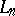be a space with an affine connection and letbe the Christoffel symbols (cf. Christoffel symbol) of the connection of. The components (coordinates) of the Riemann tensor, which is once contravariant and three times covariant, take the form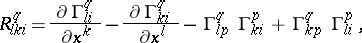whereis the symbol of differentiation with respect to the space coordinate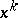,. In a Riemannian space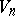with a metric tensor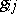, in addition to the tensor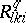the four times covariant Riemann tensor obtained by lowering the upper indexusing the metric tensoris also studied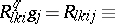Heresince the Riemannian connection (without torsion) is considered on. In an arbitrary space with an affine connection without torsion the coordinates of the Riemann tensor satisfy the first Bianchi identity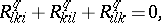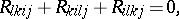i.e. the cyclic sum with respect to the first three subscripts is zero.

The Riemann tensor possesses the following properties:

1)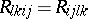;

2)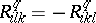;

3),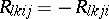;

4),, if both subscripts of one pair are identical, then the corresponding coordinate equals zero:;

5) the second Bianchi identity is applicable to the absolute derivatives of the Riemann tensor: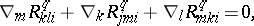whereis the symbol for covariant differentiation in the direction of the coordinate. The same identity is applicable to the tensor.

A Riemann tensor has, in all,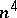coordinates,being the dimension of the space, among which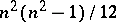are essential. Between the latter no additional dependencies result from the properties listed above.

Whenthe Riemann tensor has one essential coordinate,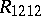; it forms part of the definition of the intrinsic, or Riemannian, curvature of the surface:(see Gaussian curvature).

The Riemann tensor was defined by B. Riemann in 1861 (published in 1876).

How to Cite This Entry:
Riemann tensor. Encyclopedia of Mathematics. URL: http://encyclopediaofmath.org/index.php?title=Riemann_tensor&oldid=15143
This article was adapted from an original article by L.A. Sidorov (originator), which appeared in Encyclopedia of Mathematics - ISBN 1402006098. See original article